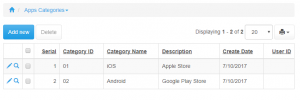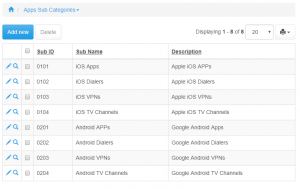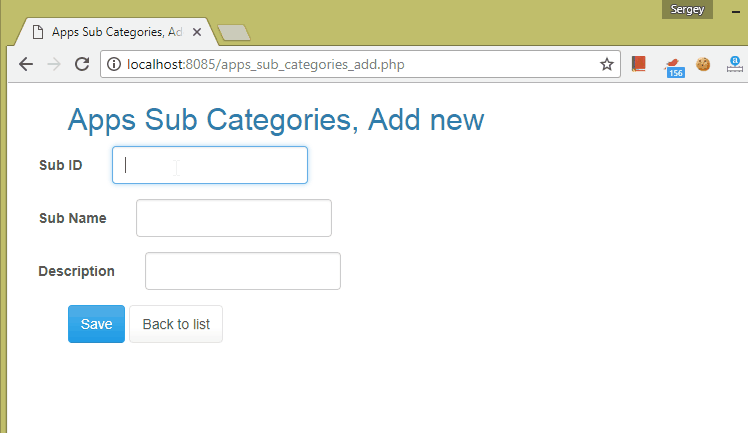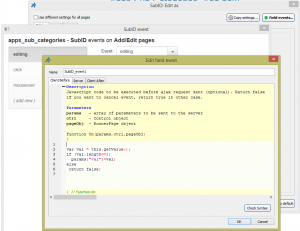# Using field events

Categories ASP.NET, PHP, Tutorials

We have two tables: categories and sub_categories. CategoryID is a two-digit category code.In sub_categories table SubID consists of two-digit category code and and two-digit subcategory code.What we need to do is to simplify adding a large number of subcategories. When user types in first two digit (category) we want to show category name underneath and also calculate the next subcategory number. We will do that using field events.

This is how it works in generated application:### Code

First we need to create Field Event for SubID field. We will use 'editing' event here which will be called every time the content of field is changed.ClientBefore:

```var val = this.getValue();
if (val.length==2)
params["val"]=val;
else
return false;
```

In ClientBefore code we check the length of entered code and only send it to server side when code is two digits long.

Server:

PHP

```\$result["catName"] = DBLookup("select CategoryName from apps_categories where CategoryID='".\$params["val"]."'");
\$sub = DBLookup("select max(substring(SubID,3,2))+1 from apps_sub_categories where left(SubID,2)='".\$params["val"]."'");
```

C#

```result["catName"] = tDAL.DBLookup("select CategoryName from categories where CategoryID='" + parameters["val"].ToString() + "'");

string sub = tDAL.DBLookup("select max(substring(SubID,3,2))+1 from sub_categories where left(SubID,2)='" + parameters["val"].ToString() + "'");

result["newCat"] = parameters["val"].ToString() + sub.ToString().PadLeft(2, '0');
```

ASP

```result("catName") = DBLookup("select CategoryName from categories where CategoryID='" & params("val") & "'")
res = DBLookup("select max(substring(SubID,3,2))+1 from sub_categories where left(SubID,2)='" & params("val") & "'")
if len(res)<2 then
res = "0" & res
end if
result("newCat") = params("val") & res
```

On Server side we pull CategoryName from categories table and also calculate next subcategory ID. We pass both category name and new subcategory code back to ClientAfter event.

ClientAfter:

```\$("#sub_tip").remove();
\$("input[id=value_SubID_1]").after("<div id='sub_tip' style='margin-top: 10px; color: blue;'>"+result["catName"]+"</div>");
ctrl.setValue(result["newCat"]);
```

In this event we remove previous tooltip with category name and add a new one. We also make it appear in blue color. Then we set the value of SubID with new subcategory code we received from the Server event.

## 2 thoughts on “Using field events”

1.Jerry says:

Very very nice. Thank you. Don’t forget C# :)

2.Wilson Santos says:

\$credito = strip_tags(\$xt->getvar(“credito_total”));
\$debito = strip_tags(\$xt->getvar(“debito_total”));
if (\$debito!=0)
//Not works assign(number_format( \$mytotal, 2 ), \$credito-\$debito);

//Works! assign(“mytotal”, \$credito-\$debito);

Anybody can help me?